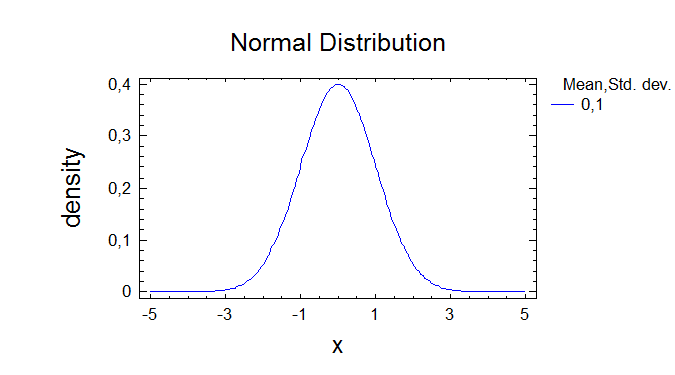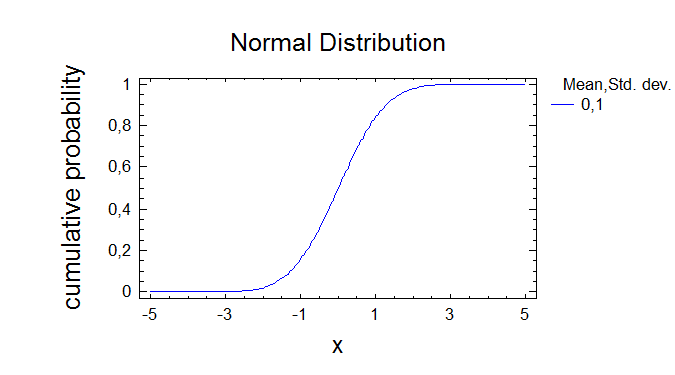# Probability Distributions. Cumulative Distribution. The Stat Advisor

### Содержание работы

Probability Distributions

Probability Distributions

------------------------Distribution: Normal

Parameters:     Mean            Std. dev.

Dist. 1      0               1

Dist. 2

Dist. 3

Dist. 4

Dist. 5

--------------This procedure allows you to analyze any of 24 probability

distributions.  Currently, the normal distribution has been selected.

You can create various plots, compute tail areas and critical values,

and generate random numbers from the selected distribution.  Up to

five sets of parameters can be specified by pressing the alternate

mouse button and selecting Analysis Options.Cumulative Distribution

----------------------Distribution: Normal

Lower Tail Area (<)

Variable      Dist. 1       Dist. 2       Dist. 3       Dist. 4       Dist. 5

0             0,5

Probability Density

Variable      Dist. 1       Dist. 2       Dist. 3       Dist. 4       Dist. 5

0             0,398942

Upper Tail Area (>)

Variable      Dist. 1       Dist. 2       Dist. 3       Dist. 4       Dist. 5

0             0,5

--------------This pane evaluates the cumulative normal distribution.  It will

calculate the tail areas for up to 5 critical values of the

distribution.  It will also calculate the probability density or mass

function.  For example, the output indicates that, for the first

distribution specified, the probability of obtaining a value less than

0,0 is 0,5.  Also, the probability of obtaining a value greater than

0,0 is 0,5.  The height of the probability density function at 0,0 is

0,398942.Probability Distributions

Probability Distributions

------------------------Distribution: Normal

Parameters:     Mean            Std. dev.

Dist. 1      0               1

Dist. 2

Dist. 3

Dist. 4

Dist. 5

--------------This procedure allows you to analyze any of 24 probability

distributions.  Currently, the normal distribution has been selected.

You can create various plots, compute tail areas and critical values,

and generate random numbers from the selected distribution.  Up to

five sets of parameters can be specified by pressing the alternate

mouse button and selecting Analysis Options.Cumulative Distribution

----------------------Distribution: Normal

Lower Tail Area (<)

Variable      Dist. 1       Dist. 2       Dist. 3       Dist. 4       Dist. 5

0             0,5

Probability Density

Variable      Dist. 1       Dist. 2       Dist. 3       Dist. 4       Dist. 5

0             0,398942

Upper Tail Area (>)

Variable      Dist. 1       Dist. 2       Dist. 3       Dist. 4       Dist. 5

0             0,5

--------------This pane evaluates the cumulative normal distribution.  It will

calculate the tail areas for up to 5 critical values of the

distribution.  It will also calculate the probability density or mass

function.  For example, the output indicates that, for the first

distribution specified, the probability of obtaining a value less than

0,0 is 0,5.  Also, the probability of obtaining a value greater than

0,0 is 0,5.  The height of the probability density function at 0,0 is

0,398942.Probability Distributions

Probability Distributions

------------------------Distribution: Normal

Parameters:     Mean            Std. dev.

Dist. 1      0               1

Dist. 2

Dist. 3

Dist. 4

Dist. 5

--------------This procedure allows you to analyze any of 24 probability

distributions.  Currently, the normal distribution has been selected.

You can create various plots, compute tail areas and critical values,

and generate random numbers from the selected distribution.  Up to

Тип:
Практика
Размер файла:
265 Kb
Скачали:
0